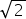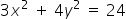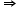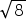Maths-
General
Easy

Question

# The sum of distances of any point on the ellipse 3 x2 + 4y2 = 24 from its foci is

## 8416none of theseHint:

## The correct answer is: 4### Given :Dividing both sides by 24, we getThe sum of the distances to any point on the ellipse (x,y) from the two foci (c,0) and (-c,0) is a constant. That constant will be 2a. If we let d1 and d2 bet the distances from the foci to the point, then d1 + d2 = 2a.d1 + d2 = 2ad1 + d2 = 2d1 + d2 = 4Thus, the sum of distances of any point on the ellipse 3 x2 + 4y2 = 24 from its foci is 4.#### With Turito Foundation.#### Get an Expert Advice From Turito.